# JP2012163412A - Physical quantity measurement instrument for rotating member - Google Patents

## Info

Publication number
JP2012163412A
JP2012163412A JP2011023149A JP2011023149A JP2012163412A JP 2012163412 A JP2012163412 A JP 2012163412A JP 2011023149 A JP2011023149 A JP 2011023149A JP 2011023149 A JP2011023149 A JP 2011023149A JP 2012163412 A JP2012163412 A JP 2012163412A
Authority
JP
Japan
Prior art keywords
physical quantity
rotating member
encoder
period
sensor
Prior art date
Legal status (The legal status is an assumption and is not a legal conclusion. Google has not performed a legal analysis and makes no representation as to the accuracy of the status listed.)
Granted
Application number
JP2011023149A
Other languages
Japanese (ja)
Other versions
JP5742265B2 (en
Inventor
Daisuke Gunji
Yasuyuki Matsuda
Ichiu Tanaka
Hiroyuki Yamamura

Original Assignee
Nsk Ltd

Priority date (The priority date is an assumption and is not a legal conclusion. Google has not performed a legal analysis and makes no representation as to the accuracy of the date listed.)
Filing date
Publication date
Application filed by Nsk Ltd, 日本精工株式会社 filed Critical Nsk Ltd
Priority to JP2011023149A priority Critical patent/JP5742265B2/en
Publication of JP2012163412A publication Critical patent/JP2012163412A/en
Application granted granted Critical
Publication of JP5742265B2 publication Critical patent/JP5742265B2/en
Active legal-status Critical Current
Anticipated expiration legal-status Critical

• 238000006073 displacement reactions Methods 0.000 claims abstract description 30
• 238000000034 methods Methods 0.000 claims description 56
• 238000005096 rolling process Methods 0.000 claims description 28
• 239000000203 mixtures Substances 0.000 description 29
• 238000003754 machining Methods 0.000 description 7
• 239000000463 materials Substances 0.000 description 3
• 238000006243 chemical reactions Methods 0.000 description 1
• 230000000694 effects Effects 0.000 description 1
• 230000004044 response Effects 0.000 description 1

## Images

•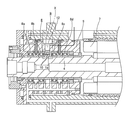•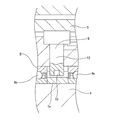•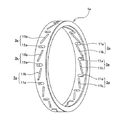•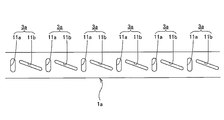•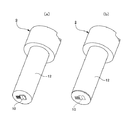•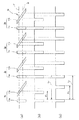•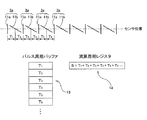•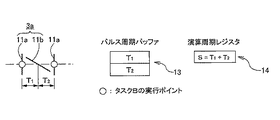•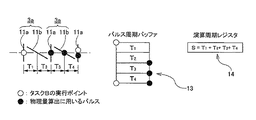•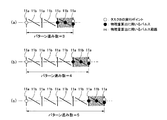•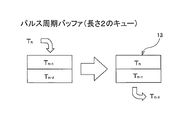•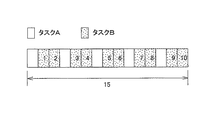•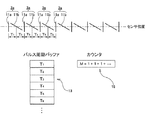•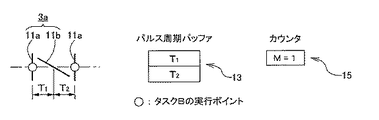•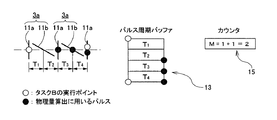•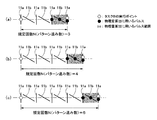•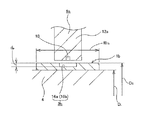•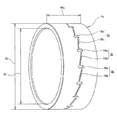•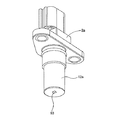•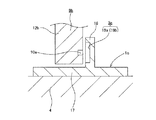•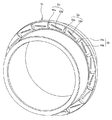•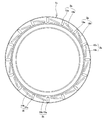•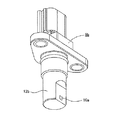•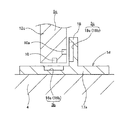••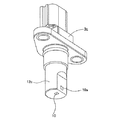•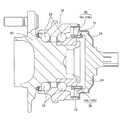•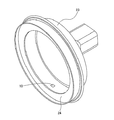•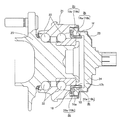•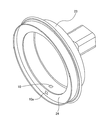•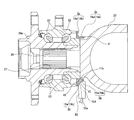•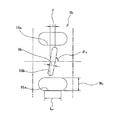•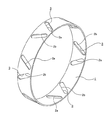•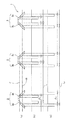## Abstract

PROBLEM TO BE SOLVED: To implement a structure for use in measurement of a physical quantity of at least one of a displacement of a rotating member and a load acting on the rotating member, which is capable of accurately measuring a rotational speed of the rotating member on the basis of an output signal of a sensor made to face a surface to be detected of an encoder 1a.SOLUTION: Among a pair of through holes 11a and 11b constituting each of characteristic change combination parts 3a and 3a provided on the surface to be detected of an encoder 1a, one through hole 11a is formed in a breadthwise direction of the surface to be detected, and the other through hole 11b is formed in a direction inclined to the breadthwise direction. A rotational speed of the rotating member is measured on the basis of a period L (L, L) between pulses generated on the basis of the through holes 11a and 11a.

## Description

The present invention provides a displacement amount of a rotating member that rotates at a high speed while receiving a high load, such as a main shaft of various machine tools such as a milling machine and a machining center, or a rotating bearing member constituting a rolling bearing unit for supporting a wheel of an automobile. And a physical quantity of at least one of the load acting on the rotating member.

For example, the spindle of a machine tool rotates at a high speed with a tool such as a blade fixed at the tip, and performs processing such as cutting on the workpiece fixed on the processing table. A spindle head (head), which is a housing that rotatably supports the spindle, moves by a predetermined amount in a predetermined direction as the workpiece is processed, and the workpiece has a predetermined size and shape. To process. In such a machining operation, it is necessary to ensure the moving speed of the spindle head in order to ensure the durability of the tool and the quality of the workpiece while ensuring the machining efficiency. That is, if the moving speed is too high, an excessive force is applied to the tool, and not only the durability of the tool is remarkably impaired, but also the surface property of the work piece is deteriorated or, Damage such as cracks may occur in the workpiece. On the contrary, when the moving speed is too slow, the processing efficiency of the workpiece is easily deteriorated.

The appropriate value of the spindle head movement speed is not constant, but varies greatly depending on the type (size) of the tool and the material and shape of the work piece. Therefore, the movement speed is kept constant while keeping the movement speed constant. It is difficult to maintain. For this reason, it is conventionally known that the moving speed is adjusted to an appropriate value by measuring the load applied to the main spindle to which the tool is fixed. That is, when a work such as cutting is performed on a workpiece with a tool, a load is applied to the tool and the main shaft to which the tool is fixed due to processing resistance. The machining resistance, and hence the load applied to the main shaft, increases as the moving speed increases, and conversely decreases as the moving speed decreases. Therefore, if the moving speed is adjusted so that the load falls within a predetermined range, the moving speed can be kept within an appropriate range.

In addition, when other conditions such as the moving speed are the same, the load increases as the cutting property (sharpness) of the tool deteriorates. Therefore, by observing the magnitude of the load in relation to the moving speed, it is possible to know that the tool has reached the end of its life, and deterioration in yield due to continuing processing with a defective tool that has reached the end of its life. Can be prevented. In addition, by continuously observing the load in association with other machining conditions such as the moving speed, it is possible to find the optimum machining conditions and lead to energy saving and long tool life. Furthermore, by continuous observation, the cause of an accident such as tool breakage can be identified.

For such a purpose, a device having a structure described in Patent Document 1, for example, is conventionally known as a device for measuring a load (cutting resistance) applied to a rotating shaft such as a main shaft of a machine tool. The load measuring apparatus described in Patent Document 1 includes a plurality of quartz piezoelectric type load sensors arranged in series with respect to the direction of load application, and supports a cutting tool based on measurement signals of these load sensors. The load (cutting resistance) applied to the fixed rotating shaft is measured. In the case of such a load measuring device described in Patent Document 1, since an expensive quartz piezoelectric load sensor is used, it is inevitable that the cost of the load measuring device as a whole increases.

On the other hand, Patent Document 2 describes an invention relating to a rolling bearing unit with a load measuring device, which includes a magnetic encoder and sensor, which can be procured at a lower cost than a quartz piezoelectric load sensor. For example, in paragraphs  to  of Patent Document 2, an encoder 1 as shown in FIG. 33 is used, and the amount of displacement in the axial direction of a rotating member that supports the encoder 1 concentrically, as a result, A technique for measuring an axial load applied to a rotating member is described.

The encoder 1 is formed by forming a magnetic metal plate such as a steel plate in a cylindrical shape, each of which is a characteristic change part, and a plurality of characteristic change combination parts 3 each consisting of a pair of through holes 2a, 2b, 3 are arranged at equal intervals in the circumferential direction. The inclination angles of the two through holes 2a and 2b constituting the characteristic change combination portions 3 and 3 with respect to the axial direction of the encoder 1 are equal in absolute value and have positive and negative signs (inclination directions). It is reversed. Such an encoder 1 is fixed to a part of a rotating member such as a main shaft of a machine tool concentrically with the rotating member. At the same time, in a state where a magnetic detection type sensor is supported on a part of a stationary member provided in a portion adjacent to the rotating member, the detection unit of the sensor is connected to the outer peripheral surface of the encoder 1 through a minute gap. And make them face each other.

In this state, when the encoder 1 rotates together with the main shaft, the detection unit of the sensor scans the outer peripheral surface of the encoder 1 which is a detected surface. Since the magnetic characteristics of the outer peripheral surface of the encoder 1 change in the circumferential direction due to the presence of the through holes 2a and 2b, the output signal of the sensor changes as the encoder 1 rotates. For example, when the detection unit of this sensor scans the chain line a position in FIG. 34 (a) on the outer peripheral surface of the encoder 1, the output signal of this sensor changes as shown in FIG. 34 (b). To do. In FIG. 34 (b), 1 is generated based on the through holes 2a and 2a on one side in the circumferential direction (left side in the figure) of the pair of characteristic change combination portions 3 and 3 adjacent in the circumferential direction. Let the period between the pair of pulses be the total period L 1 . Further, the pair of through holes 2a constituting the same characteristic change combining unit 3, the period between pulses of a pair generated based on 2b the partial period [delta] 1. When the detection unit of the sensor scans the chain line b position, the pulse cycle ratio δ 1 / L 1 , which is the ratio between the partial cycle δ 1 and the total cycle L 1 , is a relatively small value. On the other hand, when the detection unit of the sensor scans the position of the chain line in FIG. 34A, the output signal of the sensor changes as shown in FIG. The pulse cycle ratio δ 2 / L 2 , which is the ratio between the partial cycle δ 2 and the total cycle L 2 , is a relatively large value.

In this way, the pulse cycle ratio δ / L relating to the output signal of the sensor varies depending on the axial position of the outer peripheral surface of the encoder 1 (the width direction position of the detected surface) scanned by the detection unit of the sensor. And this axial position changes with the axial displacement of the rotating member which fixed the encoder. Accordingly, if software that incorporates an expression for calculating the axial displacement of the rotating member is installed in an arithmetic unit for processing the output signal of the sensor, the arithmetic unit calculates the pulse cycle ratio. Based on δ / L, the axial displacement amount of the rotating member can be calculated. Further, when the rotating member is rotatably supported by a rolling bearing to which a preload is applied, the axial displacement amount of the rotating member changes according to the magnitude of the axial load applied to the rotating member. In other words, there is a reproducible and reproducible correlation between the axial load applied to the rotating member and the axial displacement of the rotating member. And this correlation is calculated | required not only by calculation by the elastic contact theory widely known in the field of a rolling bearing but also by experiment. Therefore, if software that incorporates an equation for calculating the axial load in consideration of the correlation is installed in the calculator, the calculator calculates the pulse period ratio δ / L based on the pulse period ratio δ / L. An axial load applied to the rotating member can be calculated.

By the way, if the output signal of the sensor used in combination with the encoder 1 as described above can be used to measure the rotational speed of the rotating member such as the main shaft, it is not necessary to separately provide a device for measuring the rotational speed. Therefore, space saving and cost reduction can be achieved. Therefore, the possibility of such use will be examined. First, among each of the through holes 2a and 2b provided in the encoder 1, a plurality of through holes 2a and 2a (or 2b and 2b) each provided in the circumferential direction are arranged in the circumferential direction. Are arranged at equal intervals. In addition, the inclination angles of these through holes 2a, 2a (or 2b, 2b) in consideration of the positive and negative signs with respect to the axial direction of the encoder 1 are equal. That is, the intervals in the circumferential direction of these through holes 2a, 2a (or 2b, 2b) are equal intervals, and the size of the interval is any position in the axial direction of the encoder 1. Are also equal. Therefore, the frequency (or period) of the pulse generated based on each of the through holes 2a, 2a (or 2b, 2b) depends on the rotational speed of the rotating member regardless of the axial position of the outer peripheral surface of the encoder 1. It is proportional (or inversely proportional). For this reason, the rotational speed of the rotating member can be measured based on the frequency (or period) of the pulse.

However, the frequency (or period) of the pulse generated based on each of the through holes 2a, 2a (or 2b, 2b) depends on the variation of the axial load acting on the rotating member, apart from the rotational speed of the rotating member. Also fluctuate. In other words, each of the through holes 2 a and 2 a (or 2 b and 2 b) is inclined with respect to the axial direction of the encoder 1. In other words, the phase of the arrangement of these through holes 2a, 2a (or 2b, 2b) in the circumferential direction gradually changes in the axial direction of the encoder 1. Therefore, when the axial load acting on the rotating member (the axial position of the outer peripheral surface of the encoder 1 scanned by the detection unit of the sensor) varies, the through holes 2a, 2a (or 2b, 2b) Based on this, the phase of the generated pulse varies, and the frequency (or period) of this pulse varies accordingly. Such a variation in the frequency (or period) of the pulse is not preferable because it causes a decrease in the measurement accuracy of the rotational speed. Therefore, it is desirable to realize a structure that does not cause such inconvenience.

JP 2002-187048 A JP 2006-317420 A

In view of the circumstances as described above, the present invention is based on an output signal of a sensor used for measuring at least one physical quantity of a displacement amount of a rotating member and a load acting on the rotating member. The invention was invented to realize a structure capable of measuring the rotational speed of a member with high accuracy.

The physical quantity measuring device for a rotating member of the present invention includes a stationary member, a rotating member, an encoder, a sensor, and a calculator.
Of these, the stationary member does not rotate during use.
The rotating member is rotatably supported with respect to the stationary member by a plurality of rolling bearings each provided with a preload.
The encoder is supported and fixed to a part of the rotating member and has a detected surface concentric with the rotating member. This detected surface has a plurality of characteristic change combination portions arranged at equal intervals in the circumferential direction, and each of these characteristic change combination portions has an inclination angle that also takes into account positive and negative signs with respect to the width direction of the detected surface. Are provided with a pair of characteristic change portions different from each other in the circumferential direction of the detected surface.
Further, the sensor is supported by the stationary member in a state where the detection portion faces the detected surface. Then, each of the characteristic change units constituting each of the characteristic change combination units changes the output signal at the moment of passing through a portion of the detected surface where the detection unit faces.
Further, the computing unit processes an output signal of the sensor. Specifically, the entire period which is a period between a pair of pulses generated based on a characteristic change part on one side in the circumferential direction of a pair of characteristic change combination parts adjacent in the circumferential direction, and both of these characteristic change parts A ratio between a characteristic change part and a partial period which is a period between a pair of pulses generated on the basis of another characteristic change part arranged between these characteristic change parts with respect to the circumferential direction. Based on a certain pulse cycle ratio, each calculates a physical quantity of at least one of the displacement amount of the rotating member and the load applied to the rotating member with respect to the width direction of the detected surface.
In particular, in the physical quantity measuring device for a rotating member according to the present invention, the characteristic changing unit arranged on either side of the circumferential direction among the pair of characteristic changing units constituting each of the characteristic changing combination units. Is a non-tilt characteristic changing portion formed in the width direction of the detected surface. At the same time, the characteristic changing portion arranged on the other side in the circumferential direction is an inclined characteristic changing portion formed in a direction inclined by a predetermined angle with respect to the width direction of the detected surface.

When carrying out the present invention, preferably, as in the invention described in claim 2, it is possible to select whether or not to execute the calculation processing of the physical quantity every time the entire period elapses in the computing unit. Give function.

For this purpose, for example, as in the invention described in claim 3, each time the entire period elapses, the arithmetic unit is caused to obtain an integrated value of the entire period. At the same time, the integrated value is compared with a preset threshold value. The physical quantity calculation process is executed only when the integrated value exceeds the threshold value. In this case, the integrated value is returned to zero when the physical quantity calculation process is executed.
In this case, the configuration of the invention described in claim 4 is preferably adopted. Specifically, the physical quantity calculation process is executed once using the information acquired during the entire period after the entire period has elapsed while the rotating member is rotating at the maximum rotation speed. Based on the time required until the end, the threshold is set to a time longer than this time.

Alternatively, instead of the configuration of the invention described in claim 3 described above, for example, the configuration of the invention described in claim 5 is adopted. Specifically, the arithmetic unit is caused to measure the number of elapsed times of the entire period every time the entire period elapses. At the same time, the number of elapsed times is compared with a predetermined number of times set in advance. The physical quantity calculation process is executed only when the number of elapsed times matches the specified number of times. In this case, the number of elapsed times is reset to zero when the physical quantity calculation process is executed.
In this case, preferably, the configuration of the invention described in claim 6 is employed. Specifically, the total period of a state where the rotating member is rotating at a predetermined constant rotational speed and L S, after the elapse of this full period L S in this state during the entire period L S When the time required to complete the physical quantity calculation process once using the acquired information is T S , the specified number of times is larger than the ratio T S / L S. A natural number.

Further, when carrying out the present invention, preferably, as in the invention described in claim 7, as the encoder, a first encoder having a circumferential surface as a detected surface, and an axial side surface as a detected surface. The second encoder is provided. At the same time, as the sensor, a first sensor whose detection portion faces the detection surface of the first encoder, and a second sensor whose detection portion faces the detection surface of the second encoder. And a sensor.

Further, when the present invention is carried out, preferably, as in the invention described in claim 8, an encoder having an axial side surface as a detected surface is provided. At the same time, as the sensor, a pair of sensors is provided in which each detection unit is opposed to a portion different from each other in the circumferential direction on the detected surface of the encoder.

When carrying out the present invention, for example, as in the invention described in claim 9, the stationary member is a housing constituting a machine tool. At the same time, the rotating member is used as a main shaft constituting the machine tool.
Alternatively, as in the invention described in claim 10, the stationary member is a stationary side race ring member constituting a wheel bearing rolling bearing unit or a member constituting a part of a vehicle body. At the same time, the rotating member is a rotating side bearing ring member constituting the wheel supporting rolling bearing unit.

According to the physical quantity measuring device for a rotating member of the present invention configured as described above, the output of a sensor used for measuring at least one of the displacement amount of the rotating member and the load acting on the rotating member. Based on the signal, the rotational speed of the rotating member can be accurately measured without being affected by the change in the physical quantity.
In other words, a plurality of non-inclination characteristic changing portions are arranged at equal intervals in the circumferential direction on the detection surface of the encoder. Each of these non-inclined characteristic changing portions is formed in a direction that coincides with the width direction of the detected surface. That is, the phase of the arrangement in the circumferential direction of each of the non-tilt characteristic changing portions does not change in the width direction of the detected surface. For this reason, among the output signals of the sensor, the frequency (or period) of the pulse generated based on each non-tilt characteristic changing unit is the value of the physical quantity (the detected surface scanned by the detecting unit of the sensor). Regardless of the position in the width direction), it changes corresponding to only the rotational speed of the rotating member. Moreover, if the rotational speed is constant, the frequency (or period) of the pulse does not change even when the value of the physical quantity is changing. Therefore, based on the frequency (or period) of this pulse, the rotational speed of the rotating member can be accurately measured without being affected by the change in the physical quantity.

Further, when the present invention is implemented, if the configuration of the invention described in claims 2 to 6 is adopted, an abnormality occurs in the calculation process of the physical quantity (displacement amount, load) when the rotating member rotates at a high speed. While preventing this, the responsiveness (the number of times of calculation of the physical quantity per unit time) regarding the measurement of the physical quantity can be sufficiently secured in the entire rotation speed range of the rotating member. This point will be described in detail below.

First, the arithmetic unit constituting the physical quantity measuring device for a rotating member according to the present invention basically executes the physical quantity calculation process every time the entire period elapses. Therefore, if this total period is shortened, the responsiveness relating to the measurement of the physical quantity is improved accordingly. On the other hand, the length of this entire cycle becomes shorter (longer) as the number of characteristic change combination portions provided on the detection surface becomes larger (smaller). Therefore, if the number of characteristic change combination portions provided on the detection surface is increased, the responsiveness relating to the measurement of the physical quantity can be improved accordingly. However, the length of the whole cycle also changes depending on the rotation speed of the rotating member. That is, the length of this whole cycle becomes shorter (longer) as the rotational speed of the rotating member becomes higher (lower). Therefore, if the number of characteristic change combination portions provided on the detected surface is increased, the entire period when the rotating member rotates at high speed becomes too short, and the processing capability of the computing unit may not be able to keep up. Specifically, before the physical quantity calculation process started first is completed, the next physical quantity calculation process may be started, and this physical quantity calculation process may be executed redundantly. As a result, there is a possibility that the calculation process time of the physical quantity for each time becomes long or, if it is remarkable, the calculation process of the physical quantity cannot be performed at all. The occurrence of such an abnormality can be avoided by reducing the number of characteristic change combination portions provided on the detected surface. However, if the number of characteristic change combination portions provided on the detection surface is reduced, the length of the entire cycle in the state where the rotating member rotates at a low speed becomes long, and the responsiveness regarding the measurement of the physical quantity in this state. It becomes difficult to secure enough.

On the other hand, if the configuration of the invention described in claims 2 to 6 is adopted, for example, in the state where the rotating member rotates at a low speed, the calculation processing of the physical quantity is executed every time the entire period elapses. However, in the state where the rotating member rotates at a high speed, the calculation process of the physical quantity is not executed every time the whole period elapses, and is performed once every several times (the calculation process of the physical quantity is duplicated). Can be executed at intervals not executed). In this way, by increasing the number of characteristic change combination portions provided on the detected surface, it is possible to sufficiently ensure the responsiveness related to the measurement of the physical quantity in a state where the rotating member rotates at a low speed, and It is possible to prevent the processing capability of the computing unit from catching up with the rotating member rotating at a high speed. Therefore, in this way, while preventing the occurrence of an abnormality in the physical quantity calculation process in a state where the rotating member rotates at a high speed, the responsiveness relating to the measurement of the physical quantity can be improved with respect to all rotations of the rotating member. Sufficiently secured within the speed range.

Further, when the present invention is implemented, if the configuration of the invention described in claim 7 is adopted, the axial displacement amount of the rotating member and the rotating member are determined based on the pulse period ratio of the output signal of the first sensor. The physical quantity of at least one of the axial loads applied in the axial direction can be calculated. At the same time, the physical quantity of at least one of the radial displacement amount of the rotating member and the radial load applied to the rotating member in the radial direction can be calculated based on the pulse cycle ratio of the output signal of the second sensor.

Further, when the present invention is implemented, if the configuration of the invention described in claim 8 is adopted, the relative arrangement angle of the detection portions of the pair of sensors around the central axis of the detected surface is α degrees. In some cases, based on the pulse period ratios of the output signals of both sensors, the radial displacement amount and the radial load in two directions, each of which is a physical quantity related to the rotating member and whose crossing angle is α degrees, can be measured. it can.

[First example of embodiment]
A first example of an embodiment of the present invention corresponding to claims 1 to 4 and 9 will be described with reference to FIGS. In this example, the present invention is applied to a structure for measuring a physical quantity of at least one of an axial displacement amount of a main shaft 4 that constitutes a machine tool and an axial load applied to the main shaft 4. This is an example. The machine tool rotatably supports the spindle 4 on the inner diameter side of a housing (spindle head) 5 that is a stationary member by a multi-row rolling bearing unit 6, and the spindle 4 can be driven to rotate by an electric motor 7. It is said. Among the plurality of rolling bearings 8a to 8d constituting the multi-row rolling bearing unit 6, two rolling bearings 8a and 8b arranged near the distal end and two rolling bearings 8c and 8d arranged near the proximal end. In addition to applying contact angles opposite to each other, a preload is applied to each of the rolling bearings 8a to 8d. As a result, the main shaft 4 is supported rotatably with respect to the housing 5 in a state in which a radial load and an axial load in both directions are supported. During operation of the machine tool, a tool (not shown) fixed to the tip end portion (left end portion in FIG. 1) of the spindle 4 is pressed against the workpiece while rotating at an appropriate rotation speed. Processing such as cutting is performed. When machining is performed in this manner, a load in each direction is applied to the main shaft 4 as a reaction of pressing the tool against the workpiece. In the structure of this example, the axial displacement amount of the main shaft 4 acting on the axial load of the main shaft 4 among them (and the axial load if necessary) can be obtained. Yes.

Therefore, also in this example, as in the case of the above-described conventional structure, the combination of the encoder 1a as shown in FIGS. 3 to 4 and the single sensor 10 constituting the sensor unit 9 as shown in FIG. Is used. Of these, the encoder 1 a is fitted and fixed between the rolling bearings 8 b and 8 c constituting the multi-row rolling bearing unit 6 at the portion near the tip of the intermediate portion of the main shaft 4. The encoder 1a also serves as an inner ring spacer, is made of a magnetic metal such as steel, and has a cylindrical shape as a whole. In the encoder 1a, a plurality of characteristic change combination portions 3a and 3a are formed at equal intervals in the circumferential direction. Each of these characteristic change combination parts 3a and 3a is formed by arranging a pair of linear through holes 11a and 11b, each of which is a characteristic change part, spaced apart in the circumferential direction of the encoder 1a. In the case of this example, the through-hole 11a arranged on one side (the left side in FIG. 4) of the through-holes 11a and 11b in the circumferential direction is connected to the axial direction of the encoder 1a (see FIG. 4). The non-tilt characteristic changing portion is formed in the vertical direction in FIG. At the same time, the through hole 11b, which is also arranged on the other side (right side in FIG. 4) in the circumferential direction, has a predetermined angle (preferably about 45 to 85 degrees, preferably in a predetermined direction with respect to the axial direction of the encoder 1a. More preferably, the inclined characteristic changing portion is formed in a direction inclined by about 60 to 83 degrees, more preferably about 75 to 81 degrees.

The sensor unit 9 is formed by embedding the sensor 10 at the tip of a holder 12 made of synthetic resin. The sensor 10 has an output signal that changes based on the presence of the through holes 11a and 11b formed in the encoder 1a, and includes a Hall IC, a Hall element, an MR element, a GMR element, and the like that constitute a detection unit. And a permanent magnet. Such a sensor unit 9 is supported and fixed to the housing 5 in a state where the detection portion of the sensor 10 is close to and opposed to the outer peripheral surface of the encoder 1a, which is a detection target surface.

Also in the case of the physical quantity measuring device for a rotating member of this example having the above-described configuration, when the encoder 1a rotates together with the main shaft 4 as in the case of the conventional structure described above, the detection unit of the sensor 10 is detected. The outer peripheral surface of the encoder 1a, which is a surface, is scanned. Since the magnetic characteristics of the outer peripheral surface of the encoder 1a change in the circumferential direction due to the presence of the through holes 11a and 11b, the output signal of the sensor 10 changes as the encoder 1a rotates. For example, when the detection unit of the sensor 10 scans the position of the chain line a in FIG. 6A on the outer peripheral surface of the encoder 1a, the output signal of the sensor 10 is as shown in FIG. 6B. To change. In FIG. 6B, 1 is generated based on the through-holes 11a and 11a on one side in the circumferential direction (left side in FIG. 6) of the pair of characteristic change combination portions 3a and 3a adjacent in the circumferential direction. Let the period between the pair of pulses be the total period L 1 . Further, the pair of through holes 11a constituting the same characteristic change combination unit 3a, the period between pulses of a pair generated based on 11b and sub-periods [delta] 1. When the detection unit of the sensor 10 scans the chain line a position, the pulse period ratio δ 1 / L 1 , which is the ratio between the partial period δ 1 and the total period L 1 , is a relatively small value. . On the other hand, when the detection unit of the sensor 10 scans the position of the chain line B in FIG. 6A, the output signal of the sensor 10 changes as shown in FIG. 6C. The pulse cycle ratio δ 2 / L 2 , which is the ratio between the partial cycle δ 2 and the total cycle L 2 , is a relatively large value.

In the case of carrying out the present invention, a pair of characteristic change combination units 3a and 3a adjacent to each other in the circumferential direction is used as the partial period δ when obtaining the pulse period ratio. It is also possible to employ a period between pulses related to the through holes 11b and 11a. In this case, the direction in which the magnitude of the pulse period ratio changes is opposite to that described above. Further, as the total period L when the pulse period ratio is obtained, a period between pulses related to the through holes 11b and 11b of the pair of characteristic change combination parts 3a and 3a adjacent in the circumferential direction can also be adopted.

In any case, the pulse cycle ratio δ / L related to the output signal of the sensor 10 is determined by the axial position of the outer peripheral surface of the encoder 1a (the width direction position of the detected surface) scanned by the detection unit of the sensor 10. Change. With respect to the principle of obtaining the physical quantity (one or both of the displacement amount and the axial load in the axial direction) related to the main shaft 4 to which the encoder 1a is fixed by this pulse cycle ratio δ / L, the above-described FIGS. As explained. That is, when the output signal of the sensor 10 is sent to a calculator (not shown), the calculator calculates the axial direction of the spindle 4 based on the pulse cycle ratio δ / L according to a formula in software installed in advance. Obtain the displacement amount for. Further, an axial load applied to the main shaft 4 is obtained as necessary.

In the case of this example, a plurality of through holes 11a, 11a, each of which is a non-inclined characteristic changing portion, are arranged on the detected surface at equal intervals in the circumferential direction. Each of these through holes 11a, 11a is formed in a direction that coincides with the width direction of the detected surface. That is, the phase in the circumferential direction of each of the through holes 11a and 11a does not change in the width direction of the detected surface. For this reason, among the output signals of the sensor 10, the total period L, which is the period of the pulses generated based on the through holes 11a, 11a, is represented by L 1 , L shown in FIGS. As can be seen from the fact that 2 are equal to each other (L 1 = L 2 ), the physical quantity value (the position in the width direction of the detected surface scanned by the detection unit of the sensor 10) does not depend on the value. It changes corresponding to only the rotational speed of the main shaft 4. Moreover, if the rotation speed is constant, the total period L does not change even when the value of the physical quantity is changing. Accordingly, the rotational speed of the spindle 4 can be measured with high accuracy without being affected by the change in the physical quantity based on the entire period L.

In the case of this example, the arithmetic unit basically executes the physical quantity calculation process every time the entire period L elapses. However, if this basic is thoroughly implemented, the processing capacity of the computing unit will not be able to catch up when the total period L becomes shorter as the rotational speed of the spindle 4 becomes higher, and the physical quantity that has started to be executed first. Before the calculation process is completed, the next physical quantity calculation process is started, and this physical quantity calculation process may be executed in duplicate. As a result, there is a possibility that the calculation process time of the physical quantity for each time becomes long or, if it is remarkable, the calculation process of the physical quantity cannot be performed at all. Therefore, in order to avoid the occurrence of such an abnormality, in this example, the computing unit can select whether or not to execute the physical quantity calculation process every time the entire period L elapses. It has a function. This point will be specifically described below with reference to FIGS. In the drawings used in the following description, the characteristic change pattern of the detection surface is simplified by a plurality of thick lines as shown in the upper part of FIG. Each of these bold lines is one of the circumferential side edges of each of the through holes 11a and 11b, each of which is a characteristic boundary position of the detected surface (in this example, a rising edge of a pulse) The side edges that generate

In the case of this example, the arithmetic unit executes the arithmetic processing for obtaining the physical quantity separately for task A and task B. FIG. 7 shows the processing contents of task A among them. In this task A, a pulse generated based on each of the through holes 11a and 11b is detected, and a period T i (T 1 , T 2 , T 3 , T 4 , Tb) between a pair of pulses adjacent in the circumferential direction is detected. T 5 , T 6 ... Then, the period T i is sequentially stored in the pulse period buffer 13. Further, every time a pulse generated based on each of the through holes 11a, 11a, each of which is a non-inclined characteristic portion, is detected, the total period L (T 1 + T 2 , T 3 + T 4 , T 5 + T 6 ...), And the integrated value S of the entire period L is obtained. Then, the integrated value S is stored in the calculation cycle register 14. The integrated value S stored in the calculation cycle register 14 is updated every time a new integrated value S is obtained. Further, the integrated value S stored in the calculation cycle register 14 is compared with a preset threshold value T th . Only when the integrated value S exceeds the threshold value T th (S> T th ), the execution of the task B is started. At the same time, the integrated value S stored in the calculation cycle register 14 is returned to zero. That is, the integrated value S of the entire period L is returned to zero. The threshold T th is a time set to prevent the task B from being executed repeatedly. How to set the threshold value T th will be described later.

In the task B, the physical quantity is calculated using the latest information stored in the pulse cycle buffer 13. Specifically, the pulse cycle ratio δ / L (partial cycle δ) is obtained using the cycle T n stored last in the pulse cycle buffer 13 and the cycle T n−1 stored immediately before the cycle T n. = T n-1 , total period L = T n-1 + T n ). Then, the physical quantity is calculated based on the pulse cycle ratio δ / L.

The pattern advance number increases stepwise as the rotational speed of the main shaft 4 increases. 10A to 10C show execution points of the task B when the pattern advance number is 3 to 5. FIG. In any case, in the case of this example, the task B always stores the last cycle T n stored in the pulse cycle buffer 13 and the previous one, regardless of the value of the pattern advance number. Using the cycle T n−1 , the pulse cycle ratio δ / L (partial cycle δ = T n−1 , total cycle L = T n−1 + T n ) is obtained. Therefore, the same mathematical formula for calculating the physical quantity based on the pulse cycle ratio δ / L can always be used regardless of the value of the pattern advance number.

In the case of this example, out of the cycles T i stored in the pulse cycle buffer 13, the two cycles T n and T n immediately before the execution of the task B are used for processing in the task B. Only -1 . For this reason, it is necessary to ensure the length of the pulse cycle buffer 13 to be 2 or more. If this condition is satisfied, a finite-length queue having a length of 2 as shown in FIG. In the task A, every time a pulse generated based on each of the through holes 11a and 11b is detected, some process (at least the process of obtaining the period T i between the pulses and storing it in the pulse period buffer 13). )I do. For this reason, the processing time of the task A must be shorter than the cycle T i (shortest pulse cycle T min ) between the pulses when the main shaft 4 rotates at the maximum rotation speed. On the other hand, the processing time of the task A is determined by the processing capability of the arithmetic unit actually used. Therefore, it is necessary to determine the circumferential interval between the through holes 11a and 11b arranged on the detection surface so that the shortest pulse period Tmin is longer than that.

The method of setting the threshold value T th as described above is generalized. First, the processing time of the task A and T A, the normalized minimum pulse period normalized by T A and T min_A (T min_A> 1) , the normalization task B processing time normalized by T A T B_A Then, the threshold value T th is a value that satisfies the following expression (1).
T th > T A · RoundUp {T B — A / (T min — A −1)} −−−−− (1)
RoundUp (x) in the equation (1) means the smallest integer larger than x. When the threshold value T th is a value satisfying the equation (1) and the integrated value S of the entire period L by the task A exceeds the threshold value T th (S> T th ), the task B is If executed, this task B will not be executed redundantly.

Further, from the viewpoint of sufficiently ensuring the responsiveness related to the measurement of the physical quantity, the threshold value T th is calculated based on the equation (1) (when the arithmetic unit is equipped with a multiprocessor or a multicore processor, Among the values satisfying th > T B ), it is preferable to set the value as small as possible.

As described above, in the case of the physical quantity measuring device for a rotating member of this example, the calculation of the physical quantity is performed when the rotational speed of the spindle 4 is relatively low and the total period L exceeds the threshold value T th. The process (the task B) is executed every time the entire period L elapses. On the other hand, as the rotational speed of the spindle 4 increases, in the state where the total period L is equal to or less than the threshold value T th , the physical quantity calculation process is performed every time the total period L elapses. Is not executed, and is executed once every several times (interval where the physical quantity calculation process is not executed redundantly). For this reason, by increasing the number of characteristic change combination parts 3a, 3a provided on the detected surface sufficiently so that the processing time of the task A does not become shorter than the shortest pulse period, the spindle 4 can be operated at high speed. It is possible to sufficiently ensure the responsiveness relating to the measurement of the physical quantity in the entire rotational speed range of the spindle 4 while preventing the occurrence of abnormality in the calculation process of the physical quantity in the rotating state.

The processor installed in the arithmetic unit constituting the present invention is not limited to a relatively inexpensive single processor, but may be a relatively expensive multiprocessor or multicore processor. However, even if any processor is mounted, the above-described effects can be obtained, so that a structure with high cost performance can be realized by mounting a relatively inexpensive single processor.

[Second Example of Embodiment]
A second example of the embodiment of the present invention corresponding to claims 1, 2, 5, 6, and 9 will be described with reference to FIGS. In the case of this example, only the configuration of the arithmetic unit is slightly different from the case of the first example of the above-described embodiment. That is, in the case of this example as well, as in the case of the first example described above, the computing unit equipped with a single processor has a physical quantity (a displacement amount in the axial direction, with the spindle 4 (see FIG. 1) rotating at high speed). In order to prevent the calculation processing of the axial load) from being performed repeatedly, a function is provided that can select whether or not to execute the physical quantity calculation processing every time the entire period L elapses. However, in the case of this example, the specific content of this function is slightly different from the case of the first example described above. This point will be specifically described below.

First, the common points with the case of the first example described above will be described. The arithmetic unit executes the arithmetic processing for obtaining the physical quantity separately for task A and task B. In task A, as shown in FIG. 13, a pulse generated based on each of the through holes 11a and 11b arranged on the detection surface is detected, and a period T between a pair of pulses adjacent in the circumferential direction is detected. i (T 1 , T 2 , T 3 , T 4 , T 5 , T 6 ...) is obtained. Then, the period T i is sequentially stored in the pulse period buffer 13. In the task B, the physical quantity is calculated using the latest information stored in the pulse cycle buffer 13. Specifically, the pulse cycle ratio δ / L (partial cycle δ) is obtained using the cycle T n stored last in the pulse cycle buffer 13 and the cycle T n−1 stored immediately before the cycle T n. = T n-1 , total period L = T n-1 + T n ). Then, the physical quantity is calculated based on the pulse cycle ratio δ / L. About the above point, it is the same as that of the case of the 1st example mentioned above.

Next, the difference from the case of the first example described above will be described. In the case of this example, in the task A, in addition to executing the above-described processing, each is a non-gradient characteristic portion. Every time a pulse generated based on each of the through holes 11a, 11a is detected, that is, every time the whole period L elapses, the number M of elapsed times of the whole period L is measured. Specifically, the count value M of the counter 15 representing the elapsed number M is incremented by one. At the same time, this elapsed number (count value) M is compared with a predetermined number N set in advance. The execution of the task B is started only when the number of elapsed times (count value) M matches the specified number of times N (M = N). At the same time, the number of elapsed times (count value) M is returned to zero. The specified number N is a natural number set to prevent the task B from being executed repeatedly. How to set the specified number N will be described later.

As another example, FIGS. 16A to 16C show the execution points of the task B when the specified number N (the number of pattern advancements) is 3 to 5. FIG. In any case, in the case of this example, as in the case of the first example described above, the task B is always stored last in the pulse period buffer 13 regardless of the value of the prescribed number N. Using the cycle T n and the cycle T n−1 stored immediately before it, the pulse cycle ratio δ / L (partial cycle δ = T n−1 , total cycle L = T n−1 + T n ) Therefore, the same mathematical formula for calculating the physical quantity based on the pulse cycle ratio δ / L can always be used regardless of the value of the pattern advance number.

For example, when the steady rotational speed is relatively low, the total period L S is 30 unit hours, and the time T S is 11 unit hours, the value of these ratios T S / L S is about 0. Therefore, the prescribed number N is set to a natural number of 1 or more. Further, when the steady rotational speed is relatively high, the total period L S is 10 unit hours, and the time T S is 13 unit hours, the value of these ratios T S / L S is 1. Therefore, the prescribed number N is set to a natural number of 2 or more. Further, when the steady rotational speed is higher, the total period L S is 6 unit hours, and the time T S is 15 unit hours, the value of these ratios T S / L S is 2.5. Therefore, the specified number N is set to a natural number of 3 or more. If the prescribed number N is set as described above, the task B will not be executed repeatedly while the spindle 4 is rotating at a predetermined steady rotational speed.

When the arithmetic unit is equipped with a multiprocessor or a multicore processor, the task A and the task B can be executed independently of each other (in parallel). Therefore, in this case, the time T S in the conditional expression {N (natural number)> T S / L S } to be satisfied by the specified number N is the processing time T B of the task B.
In any case, from the viewpoint of sufficiently ensuring the responsiveness related to the measurement of the physical quantity, the prescribed number N is set to the smallest natural number among the natural numbers larger than the value of the ratio T S / L S. preferable.

Further, in the case of this example, the calculation unit of the computing unit related to the circumferential pitch of each of the through holes 11a, 11a, each of which is a non-inclined characteristic portion, and the time T S related to the entire period L S. Processing power is known. Therefore, as long as the steady rotational speed of the main spindle 4 is determined, a value to be set as the specified number N can be obtained before the operation of the machine tool is started. In the case of carrying out this example, the operation for obtaining the value to be set as the specified number N may be performed by the operator of the machine tool, or may be performed at the steady rotational speed input to the control unit of the machine tool. Based on this, the control unit may perform this. In addition, the operation of inputting the specified number of times N to the arithmetic unit may be performed by the operator via an operation panel or the like of a machine tool, or may be performed by the control unit.

As described above, in the case of the physical quantity measuring device for a rotating member of this example, when the rotational speed of the main shaft 4 is relatively low and the specified number N is set to 1, the physical quantity calculation process ( The task B) is executed every time the whole period L elapses. On the other hand, when the rotational speed of the spindle 4 is relatively high and the prescribed number N is set to 2 or more, the physical quantity calculation process should be executed every time the whole period L elapses. Instead, it is executed once every N times (an interval at which the physical quantity calculation process is not repeated). For this reason, the number of characteristic change combination portions 3a and 3a provided on the detected surface is set so that the processing time of the task A is the shortest pulse period (period T i between pulses in a state where the spindle 4 rotates at the maximum rotation speed ). ), The response to the measurement of the physical quantity is reduced while preventing the occurrence of an abnormality in the calculation process of the physical quantity in a state where the spindle 4 rotates at a high speed. It can be sufficiently secured in the entire rotational speed range of the main shaft 4. Other configurations and operations are the same as those in the first example of the embodiment described above.

[Third example of embodiment]
FIGS. 17 to 19 show a third example of the embodiment of the invention corresponding to claims 1 to 6 and 9. In the case of this example, the plurality of characteristic change combination portions 3b and 3b provided on the outer peripheral surface of the encoder 1b, which is the detection surface, are each in the axial direction that is the width direction of the detection surface, which is a characteristic change portion. A linear groove 16a formed in a distorted manner and a groove 16b formed in a direction inclined by a predetermined angle in a predetermined direction with respect to the axial direction are spaced apart in the circumferential direction of the encoder 1b. Become. As described above, the other configurations and functions are the same as those described above except that the characteristic change portions are not the through holes but the concave grooves 16a and 16b, and the shape of the holder 12a constituting the sensor unit 9a is slightly different. This is the same as in the case of the first example or the second example.

[Fourth Example of Embodiment]
20 to 23 show a fourth example of the embodiment of the invention corresponding to claims 1 to 6 and 9. FIG. In the case of this example, the encoder 1c externally fitted and fixed to the main shaft 4 includes a cylindrical portion 17 and an annular portion 18 provided at a portion closer to one axial end (right end in FIG. 20) of the outer peripheral surface of the cylindrical portion 17. With. A plurality of characteristic change combination portions 3c and 3c are formed at equal intervals in the circumferential direction on one side surface (the left side surface in FIG. 20) of the annular portion 18 which is a detected surface. Each of these characteristic change combination parts 3c and 3c is a characteristic change part, and is a linear concave groove 19a formed in the radial direction that is the width direction of the detected surface, and a predetermined value with respect to this radial direction. It comprises a linear groove 19b formed in a direction inclined by a predetermined angle in the direction. Further, in a state where the sensor unit 9b is supported and fixed to the housing 5 (see FIG. 1), the detection portion of the sensor 10a embedded in the tip portion of the synthetic resin holder 12b constituting the sensor unit 9b is used as the ring. The one side of the portion 18 is made to face and face each other.

In the case of the physical quantity measuring device for a rotating member of the present example configured as described above, based on the pulse period ratio δ / L of the output signal of the sensor 10a generated when the main shaft 4 rotates, the main shaft 4 The physical quantity of at least one of the radial displacement amount and the radial load applied to the main shaft 4 can be calculated. Other configurations and operations are the same as those in the first example or the second example of the embodiment described above.

[Fifth Example of Embodiment]
24 to 26 show a fifth example of the embodiment of the invention corresponding to claims 1 to 7 and 9. FIG. In the case of this example, the encoder 1d has a detected surface having the same configuration as the detected surface of the encoder 1b (see FIGS. 17 to 18) of the third example of the embodiment described above on the outer peripheral surface of the cylindrical portion 17a. A detected object having the same configuration as that of the detected surface of the encoder 1c (see FIGS. 20 to 22) of the fourth example of the above-described embodiment is provided on one side surface (the left side surface in FIG. 24) of the annular portion 18. Each surface is provided. Of the pair of sensors 10 and 10a embedded in the tip of the holder 12c made of synthetic resin constituting the sensor unit 9c with the sensor unit 9c supported and fixed to the housing 5 (see FIG. 1), The detection part of one sensor 10 is provided on the detection surface provided on the outer peripheral surface of the cylindrical part 17a, and the detection part of the other sensor 10a is provided on the detection surface provided on one side of the annular part 18, respectively. Closely opposed.

In the case of the physical quantity measuring device for a rotating member of the present example configured as described above, based on the pulse cycle ratio δ / L of the output signal of the one sensor 10, the amount of displacement in the axial direction of the main shaft 4 and A physical quantity of at least one of the axial loads applied to the main shaft 4 can be calculated by a first computing unit (not shown). Further, based on the pulse cycle ratio δ / L of the output signal of the other sensor 10a, the physical quantity of at least one of the radial displacement amount of the main shaft 4 and the radial load applied to the main shaft 4 is illustrated. It can be calculated by a second computing unit that does not. In this example, the one sensor 10 is the first sensor described in claim 7, the other sensor 10a is the second sensor, and the cylindrical portion 17a is the same. The ring portion 18 corresponds to one encoder, and also corresponds to the second encoder. Other configurations and operations are the same as those in the first example or the second example of the embodiment described above.

[Sixth Example of Embodiment]
27 to 28 show a sixth example of the embodiment of the invention corresponding to claims 1 to 4 and 10. In this example, a physical quantity of at least one of an axial displacement amount of the hub 20 that is a rotation side bearing ring member that constitutes a rolling bearing unit for supporting a wheel of an automobile and an axial load applied to the hub 20 is measured. This is an example in which the present invention is applied to a specific structure. The physical quantity related to the hub 20 is, for example, an anti-lock brake system (ABS), a traction control system (TCS), an electronically controlled vehicle stability control system (ESC) that is used to ensure the running stability of the automobile. ) Etc. can be used for more advanced control of the vehicle travel stabilization device.

The wheel-supporting rolling bearing unit does not rotate in a state where it is supported and fixed to a suspension device in use, and rotates together with the wheel while being supported and fixed to the inner diameter side of the outer ring 21 which is a stationary side race ring member. The hub 20 is rotatably supported via a plurality of rolling elements 22 and 22 provided in a double row. A preload is applied to each of the rolling elements 22 and 22 together with a contact angle of the rear combination type.

In the case of the present example, the inner end of the hub 20 in the axial direction (“inside” with respect to the axial direction means the center in the width direction of the vehicle in the assembled state in the automobile, and is the right side in FIGS. 27 to 31 that are outside in the width direction of the vehicle in the assembled state is referred to as “outside” in the axial direction. The same applies to the entirety of the present specification). A cylindrical encoder 1e having the same configuration as the encoder 1b of the example (see FIGS. 17 to 18) is fitted and fixed concentrically with the hub 20. In addition, a synthetic resin holder 24 is held and fixed inside a metal plate cover 23 that closes the axially inner end opening of the outer ring 21, and the detection unit of the sensor 10 embedded in a part of the holder 24. Is close to and opposed to the outer peripheral surface of the encoder 1e, which is the detected surface.

In the case of the rotating member physical quantity measuring apparatus of the present example configured as described above, the axial displacement amount of the hub 20 based on the pulse cycle ratio δ / L of the output signal of the sensor 10, and the hub The physical quantity of at least one of the axial loads applied to 20 can be calculated by a calculator (not shown). Other configurations and operations are the same as those of the first example of the embodiment described above.

[Seventh example of embodiment]
29 to 30 show a seventh example of the embodiment of the invention corresponding to claims 1 to 4, 7 and 10. In the case of this example, an encoder 1f having the same configuration as that of the encoder 1d of the fifth example of the embodiment described above (see FIGS. 24 to 25) is concentric with the hub 20 at the inner end of the hub 20 in the axial direction. The outer fitting is fixed. A synthetic resin holder 24 is held and fixed inside a metal plate cover 23 that closes the axially inner end opening of the outer ring 21, and a part of the holder 24 is opposed to the encoder 1 f. A pair of sensors 10, 10a is embedded. And the detection part of one of these sensors 10 is made to adjoin and oppose the outer peripheral surface of the cylindrical part 17b which comprises the said encoder 1f which is a to-be-detected surface. Further, the detection portion of the other sensor 10a is made to face and face one side surface (the right side surface in FIG. 29) of the circular ring portion 18 constituting the encoder 1f, which is the detection surface. In the case of this example, the proximity facing position of the detection portions of the sensors 10 and 10a with respect to the detected surfaces is the lower end position of the detected surfaces.

In the case of the physical quantity measuring device for a rotating member of this example configured as described above, the displacement amount in the axial direction of the hub 20 based on the pulse cycle ratio δ / L of the output signal of the one sensor 10; The physical quantity of at least one of the axial loads applied to the hub 20 can be calculated by a first computing unit (not shown). Further, based on the pulse cycle ratio δ / L of the output signal of the sensor 10a, at least one of the displacement amount in the vertical direction (radial direction) of the hub 20 and the radial load applied to the hub 20 in the vertical direction. Can be calculated by a second computing unit (not shown). Other configurations and operations are the same as those of the first example of the embodiment described above.

[Eighth Example of Embodiment]
FIG. 31 shows an eighth example of the embodiment of the present invention corresponding to the first to fourth, seventh, and tenth aspects. The wheel support rolling bearing unit that is the subject of the seventh example of the embodiment described above is for a driven wheel, whereas the wheel support rolling bearing unit that is the subject of this example is for a drive wheel. For this reason, a spline hole 27 is formed in the axial direction at the center portion in the radial direction of the hub 20a so that the drive shaft 26 fixed to the outer end surface of the constant velocity joint outer ring 25 is engaged with the spline. The pair of sensors 10 and 10a is embedded in a synthetic resin holder 12d constituting the sensor unit 9d. In the case of this example, the sensor unit 9d is supported and fixed to a part of a vehicle body such as a knuckle constituting a suspension device (not shown) which is a stationary member. Other configurations and operations are the same as those of the seventh example of the embodiment described above.

Although not shown in the drawings, when implementing the present invention and providing a detected surface on the side surface in the axial direction of the encoder, a pair of sensors is prepared as in the invention described in claim 8, It is also possible to adopt a configuration in which the detection units of these two sensors are close to and opposed to portions that are different from each other in the circumferential direction in the detected surface. In this case, for example, if the relative arrangement angle of the detection parts of the two sensors centered on the central axis of the detected surface is set to 90 degrees (± 10 degrees), the pulse period of the output signals of both sensors Based on the ratio, it is possible to measure a radial displacement amount and a radial load in two directions, each of which is a physical quantity related to the rotating member and whose crossing angle is 90 degrees (± 10 degrees).

When the third example of the embodiment shown in FIGS. 17 to 19 is implemented, the following specifications can be adopted as the encoder 1b (dimensions not shown in FIGS. 17 to 18). See FIG. 32 for symbols).
<Material>
S45C tempered material <whole>
Inner diameter D i : 70 mm
Outer diameter D o : 80 mm
Width (axial dimension) W 1b : 46mm
<Dove groove 16a, 16b>
Forming method: Formed by end milling Depth dimension d p : 2 mm
<Concave groove 16a>
Total: 16 (circumferentially distributed)
Length dimension L a of both side edges (straight portion): 3 mm
Width dimension W a : 2.5 mm
<Concave groove 16b>
Total: 16 (circumferentially distributed)
Inclination angle θ b with respect to the axial direction: 80.15 degrees Distance between tops of both ends Y: 0.8 mm
Width dimension W b : 1mm
<Others>
The length dimension L a (3 mm) and the distance Y (0.8 mm) are respectively the main shafts when the maximum axial load allowed by design is applied to the multi-row rolling bearing unit 6 that supports the main shaft 4. This value is set sufficiently longer than the axial displacement of 4.

DESCRIPTION OF SYMBOLS 1, 1a-1f Encoder 2a, 2b Through-hole 3, 3a-3c Characteristic change combination part 4 Main shaft 5 Housing 6 Multi-row rolling bearing unit 7 Electric motor 8a-8d Rolling bearing 9, 9a-9d Sensor unit 10, 10a Sensor 11a , 11b Through-hole 12, 12a to 12d Holder 13 Pulse period buffer 14 Calculation period register 15 Counter 16a, 16b Groove 17, 17a, 17b Cylindrical part 18 Circular part 19a, 19b Groove 20, 20a Hub 21 Outer ring 22 Rolling element 23 Cover 24 Holder 25 Outer ring for constant velocity joint 26 Drive shaft 27 Spline hole

## Claims (10)

1. A stationary member that does not rotate, a rotating member that is rotatably supported by the stationary member, and a rotating member that is supported and fixed to a part of the rotating member by a plurality of rolling bearings each preloaded. An encoder having a detection surface concentric with the sensor, a sensor supported by the stationary member in a state where the detection unit faces the detection surface, and a calculator that processes an output signal of the sensor,
The detected surface of the encoder has a plurality of characteristic change combination portions arranged at equal intervals in the circumferential direction, and each of these characteristic change combination portions also considers positive and negative signs with respect to the width direction of the detected surface. A pair of characteristic change portions having different inclination angles are provided in a state of being separated in the circumferential direction of the detected surface,
The sensor is configured to change an output signal at a moment when each of the characteristic changing units constituting the characteristic changing combination unit passes through a portion of the detected surface that faces the detecting unit,
The computing unit includes a total period which is a period between a pair of pulses generated based on a characteristic change part on one side in a circumferential direction of a pair of characteristic change combination parts adjacent in the circumferential direction, and both the characteristic change parts. A ratio between a characteristic change part and a partial period which is a period between a pair of pulses generated on the basis of another characteristic change part arranged between these characteristic change parts with respect to the circumferential direction. For a rotating member that calculates a physical quantity of at least one of a displacement amount of the rotating member and a load applied to the rotating member with respect to the width direction of the detected surface based on a pulse cycle ratio. In physical quantity measuring equipment,
Of the pair of characteristic change parts constituting each characteristic change combination part, a characteristic change part arranged on either side with respect to the circumferential direction is a non-inclined characteristic formed in the width direction of the detected surface. In addition to the change part, the characteristic change part arranged on the other side in the circumferential direction is an inclination characteristic change part formed in a direction inclined by a predetermined angle with respect to the width direction of the detected surface. A physical quantity measuring device for a rotating member.
2.   2. The physical quantity measuring device for a rotating member according to claim 1, wherein the computing unit has a function capable of selecting whether or not to execute the physical quantity calculation process every time the entire period elapses.
3.   The computing unit obtains an integrated value of the entire period every time the entire period elapses, and compares the integrated value with a preset threshold value when the integrated value exceeds the threshold value. 3. The physical quantity measuring device for a rotating member according to claim 2, wherein only the physical quantity calculation process is executed, and the integrated value is returned to zero when the physical quantity calculation process is executed.
4.   The threshold value is obtained by executing the physical quantity calculation process once using the information acquired during the entire period after the entire period has elapsed with the rotating member rotating at the maximum rotation speed. The physical quantity measuring device for a rotating member according to claim 3, wherein the time is longer than the time required until the end.
5.   The computing unit measures the number of elapsed times of the whole period every time the whole period elapses, and compares the number of elapsed times with a predetermined number of times set in advance, and the number of elapsed times coincides with the prescribed number of times. The physical quantity measurement for a rotating member according to claim 2, wherein the physical quantity calculation process is executed only when the physical quantity calculation process is performed, and the elapsed number is returned to zero when the physical quantity calculation process is executed. apparatus.
6. The predetermined number of times, the total period of a state where the rotating member is rotating at a predetermined constant rotational speed and L S, after the elapse in this state the full period L S is in the full cycle L S When the time required to complete the physical quantity calculation process once using the acquired information is T S , the natural number is larger than the value of these ratios T S / L S. Item 6. The physical quantity measuring device for a rotating member according to Item 5.
7. The encoder includes a first encoder having a circumferential surface as a detected surface and a second encoder having an axial side surface as a detected surface,
As the sensor, a first sensor whose detection unit faces the detection surface of the first encoder, and a second sensor whose detection unit faces the detection surface of the second encoder Have
The physical quantity measuring device for a rotating member according to any one of claims 1 to 6.
8. The encoder has an axial side surface as a detected surface,
The sensor includes a pair of sensors in which each detection unit is opposed to a portion different from each other in the circumferential direction in the detected surface of the encoder.
The physical quantity measuring device for a rotating member according to any one of claims 1 to 6.
9.   The physical quantity measuring apparatus for a rotating member according to any one of claims 1 to 8, wherein the stationary member is a housing constituting a machine tool, and the rotating member is a main shaft constituting the machine tool.
10.   The stationary member is a stationary side bearing ring member constituting a wheel supporting rolling bearing unit or a part constituting a vehicle body, and the rotating member constitutes a rotating side bearing ring constituting the wheel supporting rolling bearing unit. The physical quantity measuring device for a rotating member according to any one of claims 1 to 4, 7, and 8, which is a member.
JP2011023149A 2011-02-04 2011-02-04 Physical quantity measuring device for rotating member, machine tool and vehicle Active JP5742265B2 (en)

## Priority Applications (1)

Application Number Priority Date Filing Date Title
JP2011023149A JP5742265B2 (en) 2011-02-04 2011-02-04 Physical quantity measuring device for rotating member, machine tool and vehicle

## Applications Claiming Priority (1)

Application Number Priority Date Filing Date Title
JP2011023149A JP5742265B2 (en) 2011-02-04 2011-02-04 Physical quantity measuring device for rotating member, machine tool and vehicle

## Publications (2)

Publication Number Publication Date
JP2012163412A true JP2012163412A (en) 2012-08-30
JP5742265B2 JP5742265B2 (en) 2015-07-01

# Family

## Family Applications (1)

Application Number Title Priority Date Filing Date
JP2011023149A Active JP5742265B2 (en) 2011-02-04 2011-02-04 Physical quantity measuring device for rotating member, machine tool and vehicle

## Country Status (1)

JP (1) JP5742265B2 (en)

## Citations (14)

* Cited by examiner, † Cited by third party
Publication number Priority date Publication date Assignee Title
JPH0829439A (en) * 1994-07-19 1996-02-02 Toshiba Corp Turbine monitoring instrument
JPH08248049A (en) * 1995-03-08 1996-09-27 Nippondenso Co Ltd Rotation sensor
JPH08304434A (en) * 1995-04-28 1996-11-22 Nippondenso Co Ltd Revolution sensor
JPH1048233A (en) * 1996-08-06 1998-02-20 Denso Corp Rotation detector
JP2004157066A (en) * 2002-11-08 2004-06-03 Asmo Co Ltd Method and apparatus for detecting rotation speed of motor
JP2004205456A (en) * 2002-12-26 2004-07-22 Mitsutoyo Corp Counter for incremental encoder
JP2004325134A (en) * 2003-04-22 2004-11-18 Nsk Ltd Rotation support device with state detection device
JP2005345192A (en) * 2004-06-01 2005-12-15 Advics:Kk Moving velocity detector and its detection circuit
JP2007198873A (en) * 2006-01-26 2007-08-09 Nsk Ltd Rolling bearing unit with load-measuring device
JP2008157900A (en) * 2006-11-30 2008-07-10 Nok Corp Magnetic encoder
JP2008164335A (en) * 2006-12-27 2008-07-17 Mitsuba Corp Device for measuring rotational speed
JP2009198413A (en) * 2008-02-25 2009-09-03 Nsk Ltd Method and device for magnetizing encoder
JP2009250851A (en) * 2008-04-09 2009-10-29 Nsk Ltd Magnetization method of encoder
JP2010054282A (en) * 2008-08-27 2010-03-11 Nsk Ltd Device for measuring physical quantity of rotary member

## Patent Citations (14)

* Cited by examiner, † Cited by third party
Publication number Priority date Publication date Assignee Title
JPH0829439A (en) * 1994-07-19 1996-02-02 Toshiba Corp Turbine monitoring instrument
JPH08248049A (en) * 1995-03-08 1996-09-27 Nippondenso Co Ltd Rotation sensor
JPH08304434A (en) * 1995-04-28 1996-11-22 Nippondenso Co Ltd Revolution sensor
JPH1048233A (en) * 1996-08-06 1998-02-20 Denso Corp Rotation detector
JP2004157066A (en) * 2002-11-08 2004-06-03 Asmo Co Ltd Method and apparatus for detecting rotation speed of motor
JP2004205456A (en) * 2002-12-26 2004-07-22 Mitsutoyo Corp Counter for incremental encoder
JP2004325134A (en) * 2003-04-22 2004-11-18 Nsk Ltd Rotation support device with state detection device
JP2005345192A (en) * 2004-06-01 2005-12-15 Advics:Kk Moving velocity detector and its detection circuit
JP2007198873A (en) * 2006-01-26 2007-08-09 Nsk Ltd Rolling bearing unit with load-measuring device
JP2008157900A (en) * 2006-11-30 2008-07-10 Nok Corp Magnetic encoder
JP2008164335A (en) * 2006-12-27 2008-07-17 Mitsuba Corp Device for measuring rotational speed
JP2009198413A (en) * 2008-02-25 2009-09-03 Nsk Ltd Method and device for magnetizing encoder
JP2009250851A (en) * 2008-04-09 2009-10-29 Nsk Ltd Magnetization method of encoder
JP2010054282A (en) * 2008-08-27 2010-03-11 Nsk Ltd Device for measuring physical quantity of rotary member

## Also Published As

Publication number Publication date
JP5742265B2 (en) 2015-07-01

## Similar Documents

Publication Publication Date Title
JP2882105B2 (en) Method and apparatus for measuring the preload of a rolling bearing
DE10017572B4 (en) Rolling bearings with remote sensing units
US8374717B2 (en) Vibration suppressing method and vibration suppressing device for machine tool
JP4777960B2 (en) Vibration suppression device
CN101380719B (en) Vibration suppressing device for machine tool
EP1666860B1 (en) Hub unit with sensor
ES2281012T3 (en) Method and provision of sensors for the measurement of loading in a bearing with rolling elements based on the deformation of the model.
US7212927B2 (en) Load-measuring device for rolling bearing unit and rolling bearing unit for load measurement
JP5160980B2 (en) Vibration suppression method and apparatus
US8845197B2 (en) Dental machining unit with tool spindle
JP5732325B2 (en) Vibration discrimination method and vibration discrimination apparatus
JP2004003918A (en) Rolling bearing unit for supporting wheel with load-measuring device
JP2015081920A (en) Systems and methods for monitoring rotary equipment
US8517797B2 (en) Grinding machine and grinding method
CN103817352B (en) Main shaft device
EP2366977B1 (en) Determining the contact angle of a ball bearing
TWI485378B (en) Correction method of multi-component force detector for rolling resistance testing machine
US20050076721A1 (en) Vehicle wheel bearing and method for controlling a vehicle
EP1939603B1 (en) Traveling test device
US8424375B2 (en) Method for operating a sensor on or in a vehicle tire, and a sensor system
DE102011084427A1 (en) Monitoring method and monitoring device for a machine tool and machine tool
JP2009270711A (en) Wheel bearing with sensor
US8347713B2 (en) Apparatus and method for measuring dynamic rigidity of a main shaft of a machine tool
JP5436191B2 (en) Wheel bearing device with in-wheel motor built-in sensor
Xu et al. Energy based cutting force model calibration for milling

## Legal Events

Date Code Title Description
A621 Written request for application examination

Free format text: JAPANESE INTERMEDIATE CODE: A621

Effective date: 20140203

A977 Report on retrieval

Free format text: JAPANESE INTERMEDIATE CODE: A971007

Effective date: 20140807

A131 Notification of reasons for refusal

Free format text: JAPANESE INTERMEDIATE CODE: A131

Effective date: 20140819

A521 Written amendment

Free format text: JAPANESE INTERMEDIATE CODE: A523

Effective date: 20141017

TRDD Decision of grant or rejection written
A01 Written decision to grant a patent or to grant a registration (utility model)

Free format text: JAPANESE INTERMEDIATE CODE: A01

Effective date: 20150407

A61 First payment of annual fees (during grant procedure)

Free format text: JAPANESE INTERMEDIATE CODE: A61

Effective date: 20150420

R150 Certificate of patent or registration of utility model

Ref document number: 5742265

Country of ref document: JP

Free format text: JAPANESE INTERMEDIATE CODE: R150